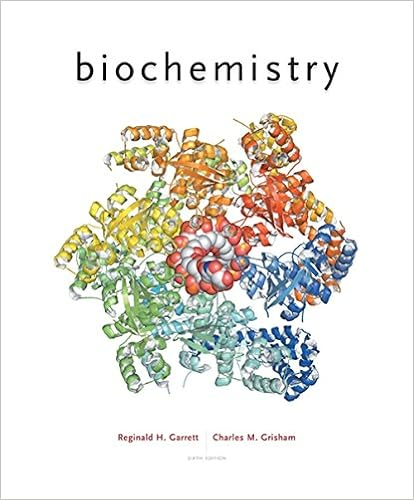# 5a what is the ph of a solution with a hydronium ion

• ahmedamr12304
• 22

This preview shows page 3 - 8 out of 22 pages.

##### We have textbook solutions for you!
The document you are viewing contains questions related to this textbook.The document you are viewing contains questions related to this textbook.
Chapter 24 / Exercise 1
Biochemistry
Garrett/GrishamExpert Verified
5.a. What is the pH of a solution with a hydronium ion concentration of 1 10 –7 mol/L? (7.0) b. What is the pH of a solution with a hydronium ion concentration of 1 10 –5 mol/L? (5.0) c. Compare the hydronium ion concentrations and pHs in (a) and (b). 2
##### We have textbook solutions for you!
The document you are viewing contains questions related to this textbook.The document you are viewing contains questions related to this textbook.
Chapter 24 / Exercise 1
Biochemistry
Garrett/GrishamExpert Verified
6.What happens to the pH of a solution when a. the hydronium ion concentration increases? b. the hydronium ion concentration decreases? Advanced (and will be tested 3 times in this course!) Follow each step for success! 7. 3.4 g of sodium hydroxide was dissociated in 2.3L of water. a. Write a dissociation equation for the above statement. b. Calculate the molar concentration of sodium hydroxide. (0.037M) c. Calculate the molar concentration of hydroxide ions. (0.037M) d. Calculate the pOH of the solution. (pOH = 1.43) e. Calculate the pH of the solution. (pH = 12.57) f. Calculate the molar concentration of hydronium ions. (2.7 x 10 -13 M)
More pH Calculations 1. What happens to the pH of a solution when a strong acid is diluted? 2. If a very acidic solution is diluted by a factor of 1000, what change would you expect in its pH? 3. What is the pH of the following solutions? Include ionization/dissociation equations. a. a 0.563 mol/L solution of nitric acid, HNO 3(aq) (0.249) b. a 9.342 × 10 -5 mol/L solution of magnesium hydroxide, Mg(OH) 2(aq) (10.2715) 4. What is the pH of a solution made by the following methods? Include dissociation/ionization equations. a. Ionizing 3.62 g of pure hydrogen iodide, HI (aq) in 2.0 L of water (1.85) b. Dissociating 7.50g of strontium hydroxide to make a 500 mL solution (13.392) 3
c. A sample of sodium hydroxide was dissolved in 1.00L water and the pOH of the solution is 1.52 at 25 o C. What mass of sodium hydroxide was needed to make this solution? (1.2g) 5. 2.8 g of hydrogen chloride gas was bubbled through a 990 mL volume of water. Write an ionization equation for the above statement. Determine the pH of the solution. (pH=1.11) 6. 0.56L of hydrogen chloride gas at 20 o C and 98kPa is bubbled through 1.5L of water. What is the resulting pH of the solution? (1.82)
Extra pH Calculations – do on separate piece of paper 1 Calculate the pH of each solution, given the following information:
2 [H 3 O + (aq) ] in cola drink is about 5.0 x 10 -3 mol/L. Calculate the approximate pH of the drink. Is the drink acidic or basic?
3 A glass of orange juice has [H 3 O + (aq) ] of 2.9 x 10 -4 mol/L. Calculate the pH of the drink. Is the drink acidic or basic?
4 [H 3 O + (aq) ] of a solution of sodium hydroxide is 6.59 x 10 -10 mol/L. Calculate the pH of the solution.
5 The concentration of a dilute solution of nitric acid, HNO 3(aq) , is 6.30 x 10 -3 mol/L. Calculate the pH of the solution.
6 A hydrobromic acid solution was made by dissolving 1.36g of the gas in enough water to make 3.50 L of solution. Determine the pH. 2.319 7 What is the [H 3 O + aq ] of a solution with a pH of: a 1.40 b 2.90 c 13.10 d 15.30 8 What is the [OH - (aq) ] of a solution with a pH of a 1.25 b 3.00 c 11.60 d 15.30 9 A solution of hydrochloric acid has a [ ] of 0.0100 mol/L. What is the [ ] of the H 3 O + (aq) ions produced? 10 What number of grams of hydroiodic acid must be dissolved in 550 mL of water in order to produce a solution with a [ ] of 1.30 mol/L? 11 Using the above solution, what is the [ ] of H 3 O + ions produced? 12 How many grams of magnesium chloride must be dissolved in 600 mL of water if the [ ] of the metallic ions is 0.400 mol/L? 13 What is the pH of the following solutions?
14 What is the pOH of the following solutions given their hydroxide ion concentrations?
•••